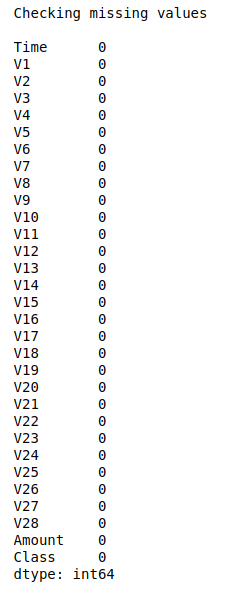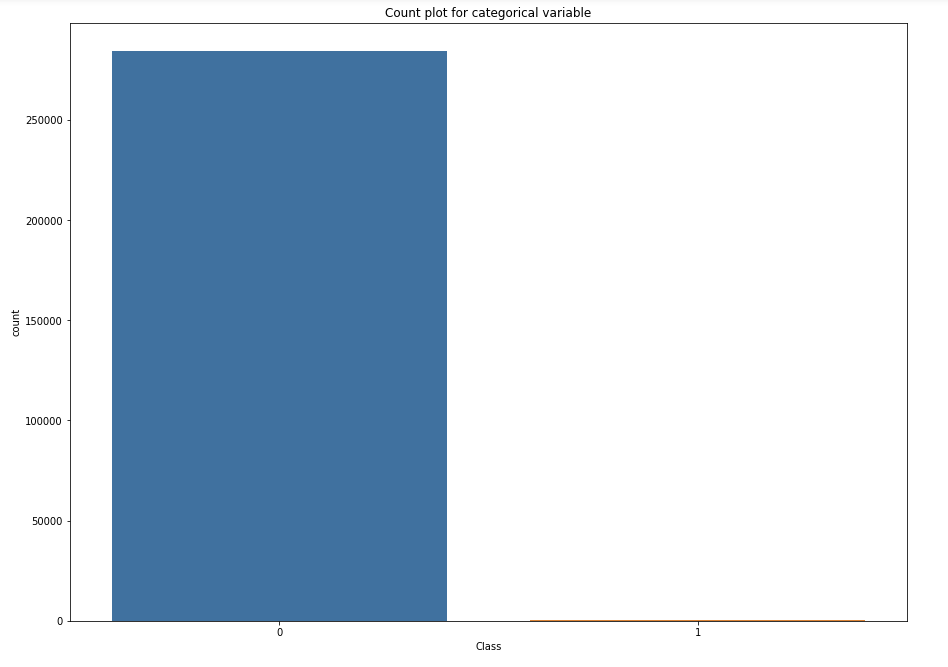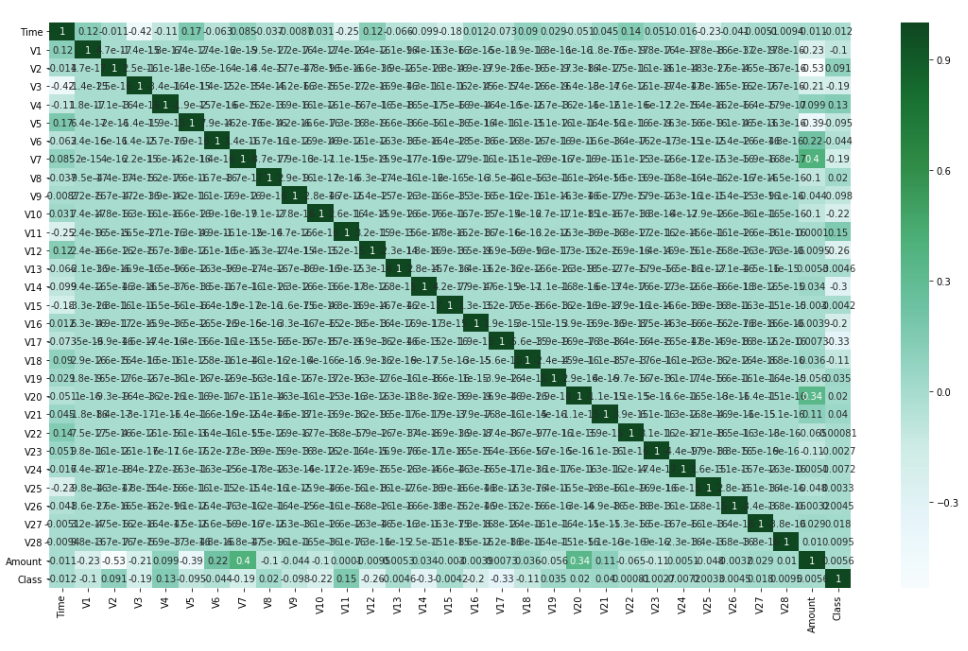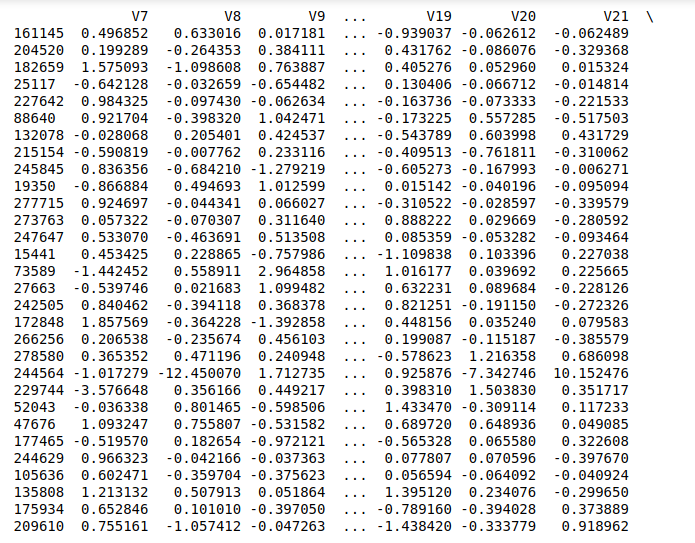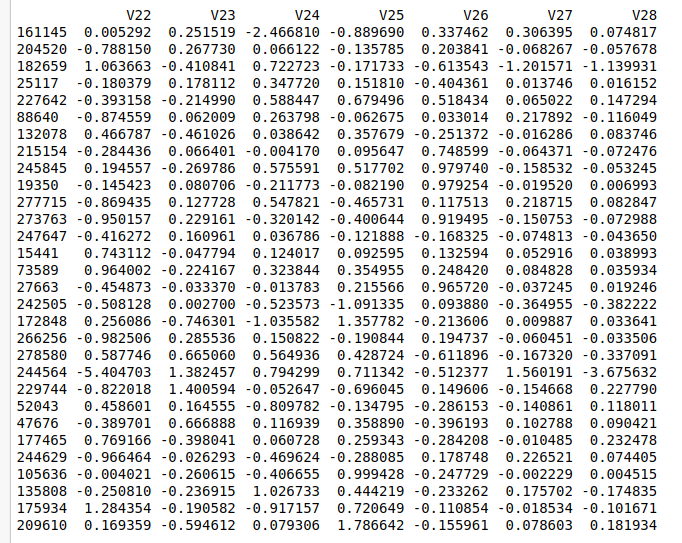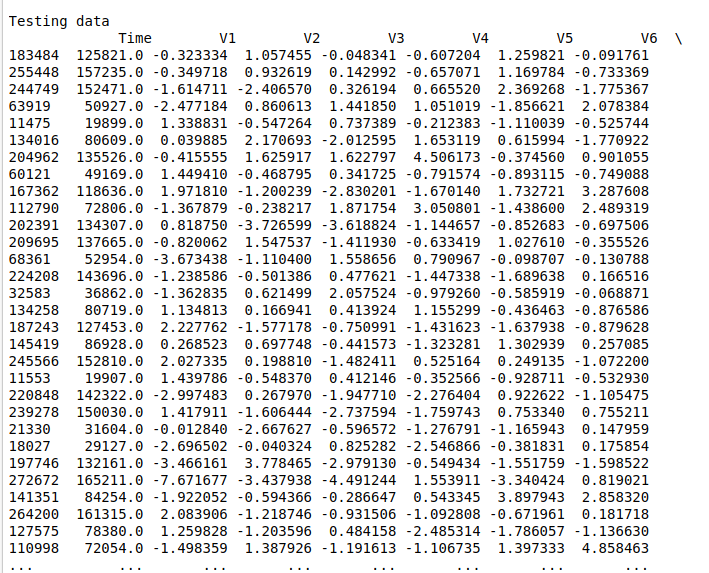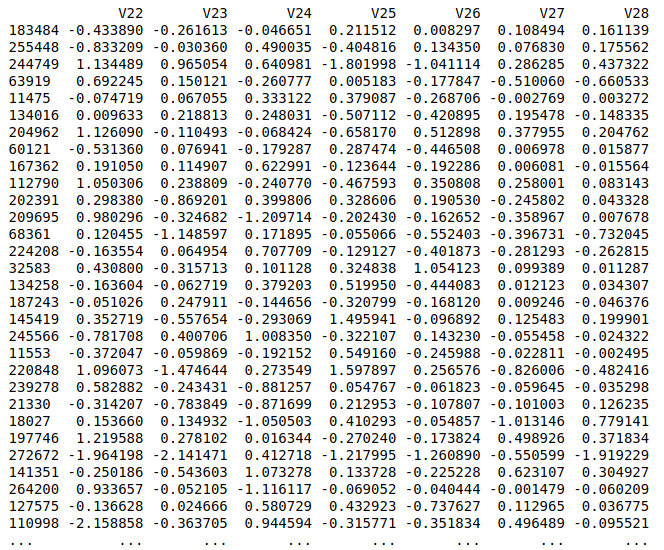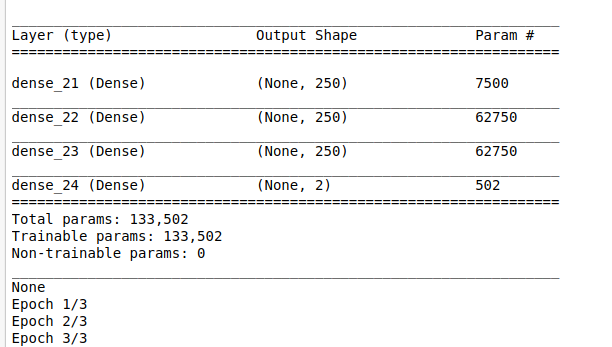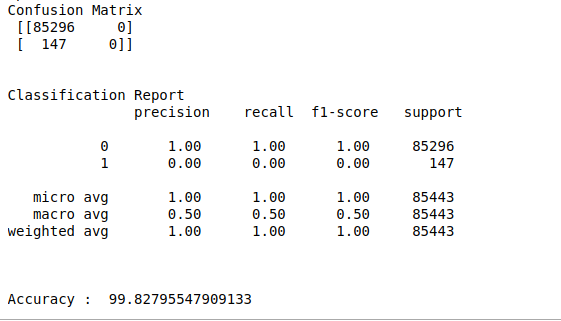• #5, First Floor, 4th Street Dr. Subbarayan Nagar Kodambakkam, Chennai-600 024 Landmark : Samiyar Madam
• pro@slogix.in
• +91- 81240 01111

### How to 0?

###### Description

To implement Deep Neural Networks model for detect fraud transaction using keras in python.

###### Input

Credit card transaction data set.

###### Output

Confusion matrix
Classification report
Accuracy score
Precision and recall

#### process:

Define the independent and dependent variable.

Build the deep neural networks.

Initiate activation and optimizer functions according to the problem.

Split the data into train and testing set.

Fit the training set into the model.

Predict the test results using DNN.

Calculate the accuracy, precision and recall.

###### Sample Code

#import necessary libraries
import time
import random
import warnings
warnings.filterwarnings(“ignore”)
import pandas as pd
import numpy as np
import matplotlib.pyplot as plt
import seaborn as sns
from keras.models import Sequential
from keras.layers import Dense
from sklearn.model_selection import train_test_split
from keras.utils import np_utils
from sklearn.metrics import classification_report, confusion_matrix

#load the sample data from sample.xlsx file

#Make it as a data frame
df = pd.DataFrame(data)

#Checking missing values
print(“Checking missing values\n”)
print(df.isnull().sum())

#Count plot for target variable
plt.rcParams[“figure.figsize”] = [15,11]
ax = sns.countplot(x=”Class”,data=df)
plt.title(“Count plot for categorical variable”)
plt.show()
print(“\n”)

#correlation plotting
plt.rcParams[“figure.figsize”] = [19,11]
corr = df.corr(method=’pearson’)
ax = sns.heatmap(corr,annot=True,cmap=’BuGn’)
plt.show()

#feature selection
X = df.iloc[:,0:29]
y = df.iloc[:,30]
#Split the data into train and testing
X_train, X_test, Y_train, Y_test = train_test_split(X, y, test_size=0.3, random_state=0)

#Print training data
print(“Training data\n”,X_train,”\n”,Y_train)
print(“\n\n”)

#Print testing data
print(“Testing data\n”,X_test)
print(“\n\n”)

#make dependent variable categorical
Y_train = np_utils.to_categorical(Y_train,num_classes=2)
Y_test = np_utils.to_categorical(Y_test,num_classes=2)

batch_size = 100

#Build Deep neural networks
n_cols_2 = X_train.shape

#create model
model = Sequential()

print(model.summary())

#Compile the model

#Here we train the Network.
model.fit(X_train, Y_train, batch_size = batch_size, epochs = 3, verbose = 5)

#Here we evaluate the model
score,acc = model.evaluate(X_test,Y_test,verbose = 2,batch_size = batch_size)
#Predict the test results
prediction = model.predict(X_test)
length = len(prediction)
y_label = np.argmax(Y_test,axis=1)
predict_label = np.argmax(prediction,axis=1)

#classification report
print(“Confusion Matrix\n”,confusion_matrix(Y_test.argmax(axis=1),prediction.argmax(axis=1)))
print(“\n”)
print(“Classification Report\n”,classification_report(Y_test.argmax(axis=1),prediction.argmax(axis=1)))
print(“\n”)
accuracy = np.sum(y_label==predict_label)/length * 100
print(“Accuracy : “,accuracy)

###### Screenshots